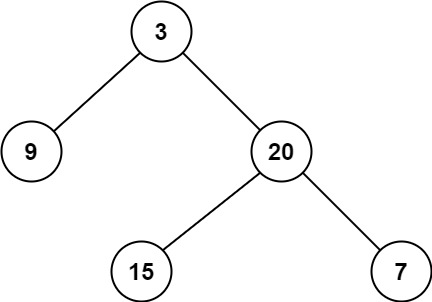# GeetCode Hub

Given a binary tree, find its minimum depth.

The minimum depth is the number of nodes along the shortest path from the root node down to the nearest leaf node.

Note: A leaf is a node with no children.

Example 1:```Input: root = [3,9,20,null,null,15,7]
Output: 2
```

Example 2:

```Input: root = [2,null,3,null,4,null,5,null,6]
Output: 5
```

Constraints:

• The number of nodes in the tree is in the range `[0, 105]`.
• `-1000 <= Node.val <= 1000`

/** * Definition for a binary tree node. * public class TreeNode { * int val; * TreeNode left; * TreeNode right; * TreeNode() {} * TreeNode(int val) { this.val = val; } * TreeNode(int val, TreeNode left, TreeNode right) { * this.val = val; * this.left = left; * this.right = right; * } * } */ class Solution { public int minDepth(TreeNode root) { } }